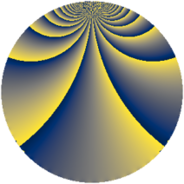# Properties

 Label 3549.2.bvLevel $3549$ Weight $2$ Character orbit 3549.bv Rep. character $\chi_{3549}(1094,\cdot)$ Character field $\Q(\zeta_{12})$ Dimension $1564$ Sturm bound $970$

# Related objects

## Defining parameters

 Level: $$N$$ $$=$$ $$3549 = 3 \cdot 7 \cdot 13^{2}$$ Weight: $$k$$ $$=$$ $$2$$ Character orbit: $$[\chi]$$ $$=$$ 3549.bv (of order $$12$$ and degree $$4$$) Character conductor: $$\operatorname{cond}(\chi)$$ $$=$$ $$273$$ Character field: $$\Q(\zeta_{12})$$ Sturm bound: $$970$$

## Dimensions

The following table gives the dimensions of various subspaces of $$M_{2}(3549, [\chi])$$.

Total New Old
Modular forms 2052 1724 328
Cusp forms 1828 1564 264
Eisenstein series 224 160 64

## Trace form

 $$1564q - 2q^{3} + 4q^{6} + 8q^{7} - 2q^{9} + O(q^{10})$$ $$1564q - 2q^{3} + 4q^{6} + 8q^{7} - 2q^{9} + 12q^{10} - 36q^{12} + 6q^{15} - 1328q^{16} + 2q^{18} + 20q^{19} + 6q^{21} + 8q^{22} - 2q^{24} - 32q^{27} - 68q^{28} - 18q^{30} - 42q^{31} + 16q^{33} + 48q^{34} + 60q^{36} - 14q^{37} - 92q^{40} + 6q^{42} + 108q^{43} + 2q^{45} + 24q^{46} + 32q^{48} + 56q^{49} + 36q^{51} - 14q^{54} + 24q^{55} - 10q^{57} - 44q^{58} + 58q^{60} - 36q^{61} - 20q^{63} + 30q^{66} + 52q^{67} + 54q^{69} + 104q^{70} - 46q^{72} + 82q^{73} - 116q^{76} - 32q^{79} - 22q^{81} - 36q^{82} - 172q^{84} - 56q^{85} - 212q^{87} - 132q^{88} - 46q^{93} + 16q^{94} + 90q^{96} - 62q^{97} + 10q^{99} + O(q^{100})$$

## Decomposition of $$S_{2}^{\mathrm{new}}(3549, [\chi])$$ into newform subspaces

The newforms in this space have not yet been added to the LMFDB.

## Decomposition of $$S_{2}^{\mathrm{old}}(3549, [\chi])$$ into lower level spaces

$$S_{2}^{\mathrm{old}}(3549, [\chi]) \cong$$ $$S_{2}^{\mathrm{new}}(273, [\chi])$$$$^{\oplus 2}$$